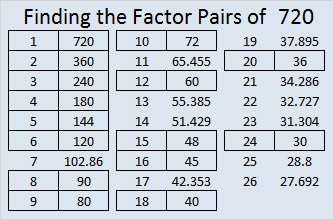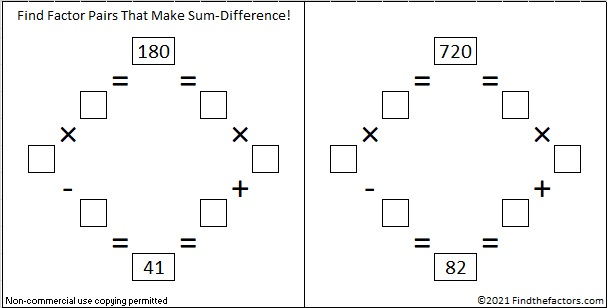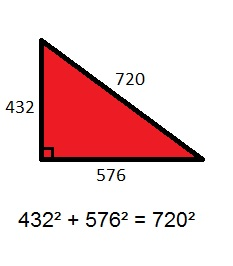# 720 Christmas Factor Trees

Contents

### Possible Christmas Dinner Choices:

Since I hosted a Christmas Eve dinner for my extended family, I thought of some of the many ways people could fill up their plates.If we made a tree diagram of all the possible dinners that could be made with exactly one item from each column, it would contain 720 lines and require quite a few pages.

The fundamental counting principle tells us the easiest way to count all those dinners is to multiply together the number of items in each column. In this case that would be 6 ⋅ 5 ⋅ 4 ⋅ 3 ⋅ 2 ⋅ 1 = 720.

6 ⋅ 5 ⋅ 4 ⋅ 3 ⋅ 2 ⋅ 1 = 720, and is usually written 6! = 720.

### Factor Trees for 720:

Making a tree diagram for 720 would be quite tedious, but making a factor tree for 720 is so easy, that I made several of them:None of those were very attractive, but here are some that are much better looking and actually look like Christmas trees:### Factors of 720:

720 has 30 factors. That’s more than any previous number!

• 720 is a composite number.
• Prime factorization: 720 = 2 x 2 x 2 x 2 x 3 x 3 x 5, which can be written 720 = (2^4) x (3^2) x 5
• The exponents in the prime factorization are 4, 2 and 1. Adding one to each and multiplying we get (4 + 1)(2 + 1)(1 + 1) = 5 x 3 x 2 = 30. Therefore 720 has exactly 30 factors.
• Factors of 720: 1, 2, 3, 4, 5, 6, 8, 9, 10, 12, 15, 16, 18, 20, 24, 30, 36, 40, 45, 48, 60, 72, 80, 90, 120, 144, 180, 240, 360, 720
• Factor pairs: 720 = 1 x 720, 2 x 360, 3 x 240, 4 x 180, 5 x 144, 6 x 120, 8 x 90, 9 x 80, 10 x 72, 12 x 60, 15 x 48, 16 x 45, 18 x 40, 20 x 36 or 24 x 30
• Taking the factor pair with the largest square number factor, we get √720 = (√144)(√5) = 12√5 ≈ 26.8328157.### Sum-Difference Puzzles:

180 has nine factor pairs. One of those pairs adds up to 41, and  another one subtracts to 41. Put the factors in the appropriate boxes in the first puzzle.

720 has fifteen factor pairs. One of the factor pairs adds up to ­82, and a different one subtracts to 82. If you can identify those factor pairs, then you can solve the second puzzle!The second puzzle is really just the first puzzle in disguise. Why would I say that?

### More about the Number 720:

Here are some other random facts about the number 720:

Because 5 is one of its factors, 720 is the hypotenuse of the Pythagorean triple 432-576-720. What is the greatest common factor of those 3 numbers? The greatest common factor is in the factor pair with the number 5. All 15 factor pairs for 720 are listed at the end of the post.The sum of the interior angles of any hexagon is 720 degrees.720 is a palindrome in three different bases:

• 5A5 in base 11; note that 5(121) + 10(11) + 5(1) = 720.
• OO in base 29; (O is 24 base 10) note that 24(29) + 24(1) = 720.
• KK in base 35; (K is 20 base 10) note that 20(35) + 20(1) = 720.

This site uses Akismet to reduce spam. Learn how your comment data is processed.